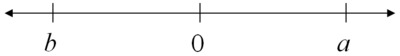# Distances on the Number Line 2

Alignments to Content Standards: 7.NS.A.1On the number line above, the numbers $a$ and $b$ are the same distance from $0$. What is $a+b$? Explain how you know.

## IM Commentary

The purpose of this task is meant to reinforce students' understanding of rational numbers as points on the number line and to provide them with a visual way of understanding that the sum of a number and its additive inverse (usually called its "opposite") is zero. Students should have lots of opportunities to represent adding specific rational numbers before they work on answering this one.

## Solution

We are given that $a$ and $b$ are the same distance from zero. However, from the above number line we can see that $a$ and $b$ are on different sides of zero. We can visualize this by representing $a$ and $b$ as directed distances on the number line:If we start at zero and move $a$ units to the right, and then move the same number of units to the left, we will be back at 0.

We can also represent this symbolically. Since $a$ and $b$ are the same distance from zero but are on opposite sides of zero, we know that they are opposites, so $b = -a$. The sum of a number and its opposite is always zero.

\begin{align} a+b=& a+(-a) \\ =& a-a \\ =& 0 \end{align}